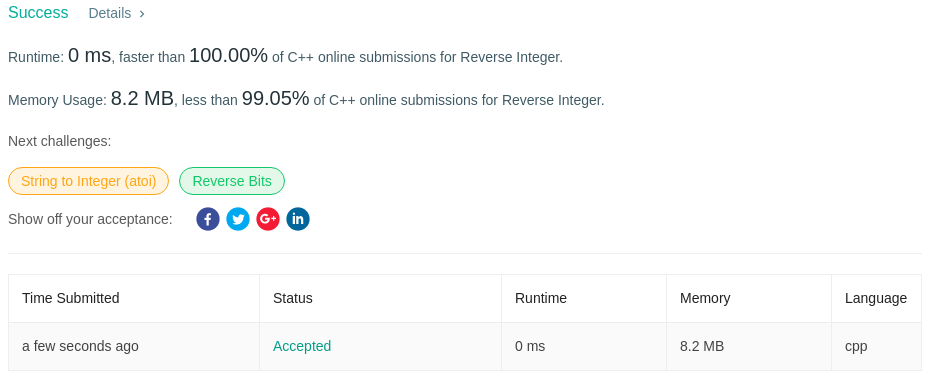Algorithm Puzzles everyday every week sometimes: Reverse Integer

## Puzzle

Puzzle from leetcode:

Given a 32-bit signed integer, reverse digits of an integer.

Example 1:

Input: 123
Output: 321
Example 2:

Input: -123
Output: -321
Example 3:

Input: 120
Output: 21

Note:
Assume we are dealing with an environment which could only store integers within the 32-bit signed integer range: [−231, 231 − 1]. For the purpose of this problem, assume that your function returns 0 when the reversed integer overflows.

## Solution

This puzzle is ez to solve if you considered possible overflow at the very beginning. I’d like to using string to do reverse process, which should be no overflow during reverse, after reversing then do overflow check. Considering that the range of int32_t is [-2147483648, 2147483647], we need to check the value after reversing is in this range or not.

Result I got:One shot pass~~